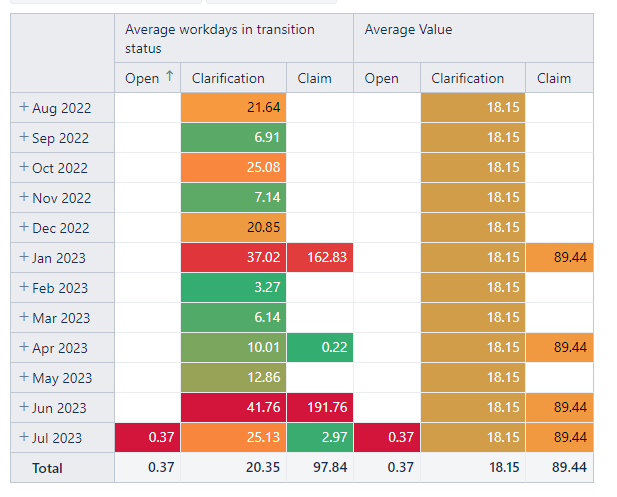# How to have the average of one column at bottom

Hello.
I have the following report:I would like to have the average value where I have the total results. It seems that the total is being calculated with all the values (including the values that are not shown in the report). For example, where I have the total ‘20.35’ set ‘18.15’. Is this possible?

Best Regards.

Hi @Lucia,

In each period (month), the “Average workdays in transition status” is based on a different set of issues that went through the particular transition status that month. And the Total row calculates the average value on all issues from Aug 2022 till Jul 2023, keeping the same principle (resulting in 20.35).

On the other hand, the value 18.15 is the average value of rows, or more specifically, the average value of average working days for each period; this value does not say anything about the issue’s average time in transition status.
Anyhow, if you want to get the average value of rows, you should add standard calcaution to the report. In the report, click on measure “Average workdays in transition status” header → Add calculated → Statistical → Average.
Here are more details on standard calculations: Add standard calculations based on a selected measure.

There is a thin chance for both results to match as each operates on a different logic.

Best,
Zane / support@eazyBI.com